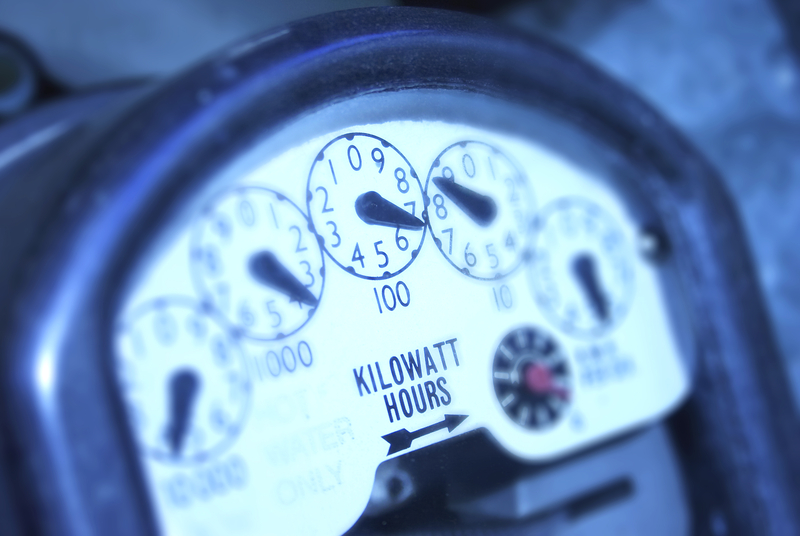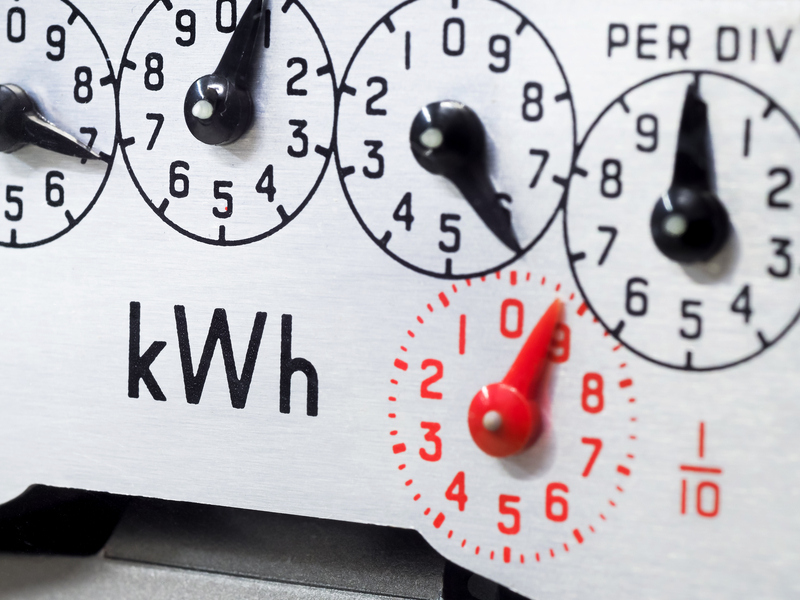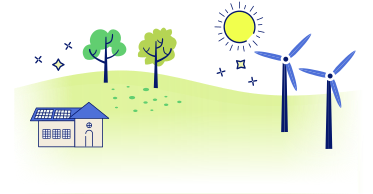# What is the Difference Between kWh vs kW? – Kilowatt vs Kilowatt-HoursThis article is your guide to the difference between kW and kWh. Have you noticed terms like kilowatt and kilowatt-hour on your energy bill? These terms may look similar, but they represent two different concepts. Kilowatt (kW) measures the power required to operate a device, while kilowatt-hour (kWh) measures the energy consumed by that device over time. In other words, kW tells you how much power you’re using at any given moment, while kWh tells you how much energy you’ve used over a certain period. In this article, we are going to take a closer look at the differences between these two units of measurement.

Here at The Energy Professor, we want to give you the information you need to not only, but to also become more energy efficient. We hope find this post helpful! And makes it easier for you to know more about kWh vs kW. Be sure to also check out our one of a kind energy savings calculator!

The Energy Professor Electricity Rate Check Tool

Contents

## What is the Difference Between kW and kWh?The difference between kilowatt and kilowatt-hours comes down to what concept of energy they measure. Kilowatt is a unit of power that measures the rate at which energy is produced and kilowatt-hour is the unit of energy that measures energy produced over time. For example, if your space heater has a power rating of 1.5 kW, it will consume 1.5 kilowatts of power for every hour in use. If you use your space heater for 4 hours, you will have used your space heater for 4 kilowatt-hours of energy in total. That number is calculated by taking the the kilowatt rating (1.5 kW) and multiplying it by the time it was used.

When you receive your electric bill, you will be billed in kWh multiplied by your local electric rate. The utility company will use Energy (kWh) = Power (kW) x Time (h) for each appliance or device you use and bill you for the total kWh you use.### What is a kW?

A kilowatt (kW) is a unit of power that measures the rate at which energy is produced or consumed. It is derived from the standard unit of power, the watt, and specifically represents 1,000 watts of power. For example, if an appliance has a power rating of 2 kW, it consumes or produces 2,000 watts of power every hour it is in use.

### What is a kWh?

A kilowatt-hour is a unit of energy commonly used to measure the amount of energy consumed or produced over a period of time. One kilowatt-hour is equal to the amount of energy used by 1-kilowatt (1 kW) appliance the operates for one hour. If you have a TV that has a rating of 3 kW, it will use 3 kWh per every hour of use.

### Why is it important to know the difference between kW and kWh?

It is important to know the difference between kW and kWh because they are very much two different measurements. Those trying to understand their electric bill might be confused if they see that they are billed in kWh, but only see their appliances in kW. Knowing the difference between kilowatts and kilowatt-hours can help you understand your electricity usage. Especially with the rising costs of electricity, it is important to analyze your energy usage and know kilowatt vs kilowatt-hour.

### What is the conversion between kW and kWh?

To convert kW into kWh, you will need this simple equation:  Energy (kWh) = Power (kW) x Time (h). You will also need the wattage of your appliance and the time it is used to convert kWh to kW.

For example, if you have a 2-kW appliance that is used for 3 hours, the energy used would be:

Energy (kWh) = 2 kW x 3 h = 6 kWh

So, the energy consumed by a 2-kW appliance for 3 hours would be 6 kilowatt-hours.

Related post: How Many Watts in One Kilowatt Hour?

## kWh vs kW FAQ### Q: Are kilowatts and kilowatt-hours the same?

A: No, kilowatts and kilowatt-hours are not the same thing. Kilowatts are the unit of power that measures the rate at which energy is produced. Kilowatt-hours is the unit of energy that measures the total power used over a period of time in kilowatts.

### Q: How many kilowatts are in a kilowatt-hour?

A: One kilowatt-hour is equal to the amount of energy that a 1-kilowatt (1 kW) appliance would use in one hour. Therefore, 1 kilowatt-hour is equivalent to 1 kilowatt (1 kW) multiplied by 1 hour (1 h), which gives 1 kilowatt-hour (1 kWh). To summarize, 1 kilowatt-hour (1 kWh) is not equivalent to any number of kilowatts, but rather it represents the amount of energy used by a 1-kilowatt appliance in 1 hour.

### Q: How do you convert kilowatts to kilowatt-hours?

A: To convert kilowatts to kilowatt-hours, all you need is the period of time the appliance is being used and the wattage of the device. To convert kW into kWh, you will need this simple equation:  Energy (kWh) = Power (kW) x Time (h). You will also need the wattage of your appliance and the time it is used to convert kWh to kW.

## Do you Need Cheaper Electricity?

If you’ve taken the time to understand the information on your bill and discovered you’re paying more than you’d like for your electricity, have you looked around for a cheaper deal? The Energy Professor has a wealth of information on ways to save on your utilities, including details of top deals that could significantly reduce your monthly or quarterly electricity bills.

We hope you found this article helpful! If you are looking for ways to increase the energy efficiency and sustainability in your home be sure to take a look at all of the latest renewable energy options in your area. The Energy Professor helps residential and small business owners find qualified energy suppliers in, Texas, Ohio, Maryland, Illinois, and Massachusetts##### Nik Chapman

Nik Chapman is the Chief Editor of The Energy Professor with lifelong passion for studying and exploring the natural environment. Nik has a Bachelor of Science in Environmental Sciences and Biology and has specialized in work with Green Energy, Renewable Energy and Environmental Justice. Nik has worked directly with small communities and nonprofits to help make environmental education more accessible for everyone. Nik currently lives in Washington State and enjoys tide pooling on the Oregon Coast and taking hikes to local waterfalls!